Subject:
Numbers and Operations
Material Type:
Lesson Plan
Level:
Middle School
6
Provider:
Pearson
Tags:
• Inequality
• Number Line
Language:
English
Media Formats:
Text/HTML

# Representing An Inequality On A Number Line# Lesson Overview

Students represent inequalities on a number line, find at least one value that makes the inequality true, and write the inequality using words.

SWD:When calling on students, be sure to call on ELLs and to encourage them to actively participate. Understand that their pace might be slower or they might be shy or more reluctant to volunteer due to their weaker command of the language.

SWD:Thinking aloud is one strategy for making learning visible. When teachers think aloud, they are externalizing their internal thought processes. Doing so may provide students with insights into mathematical thinking and ways of tackling problems. It also helps to model accurate mathematical language.

# Key Concepts

Inequalities, like equations, have solutions. An arrow on the number line—pointing to the right for greater values and to the left for lesser values—can be used to show that there are infinitely many solutions to an inequality.

The solutions to x < a are represented on the number line by an arrow pointing to the left from an open circle at a.

Example: x < 2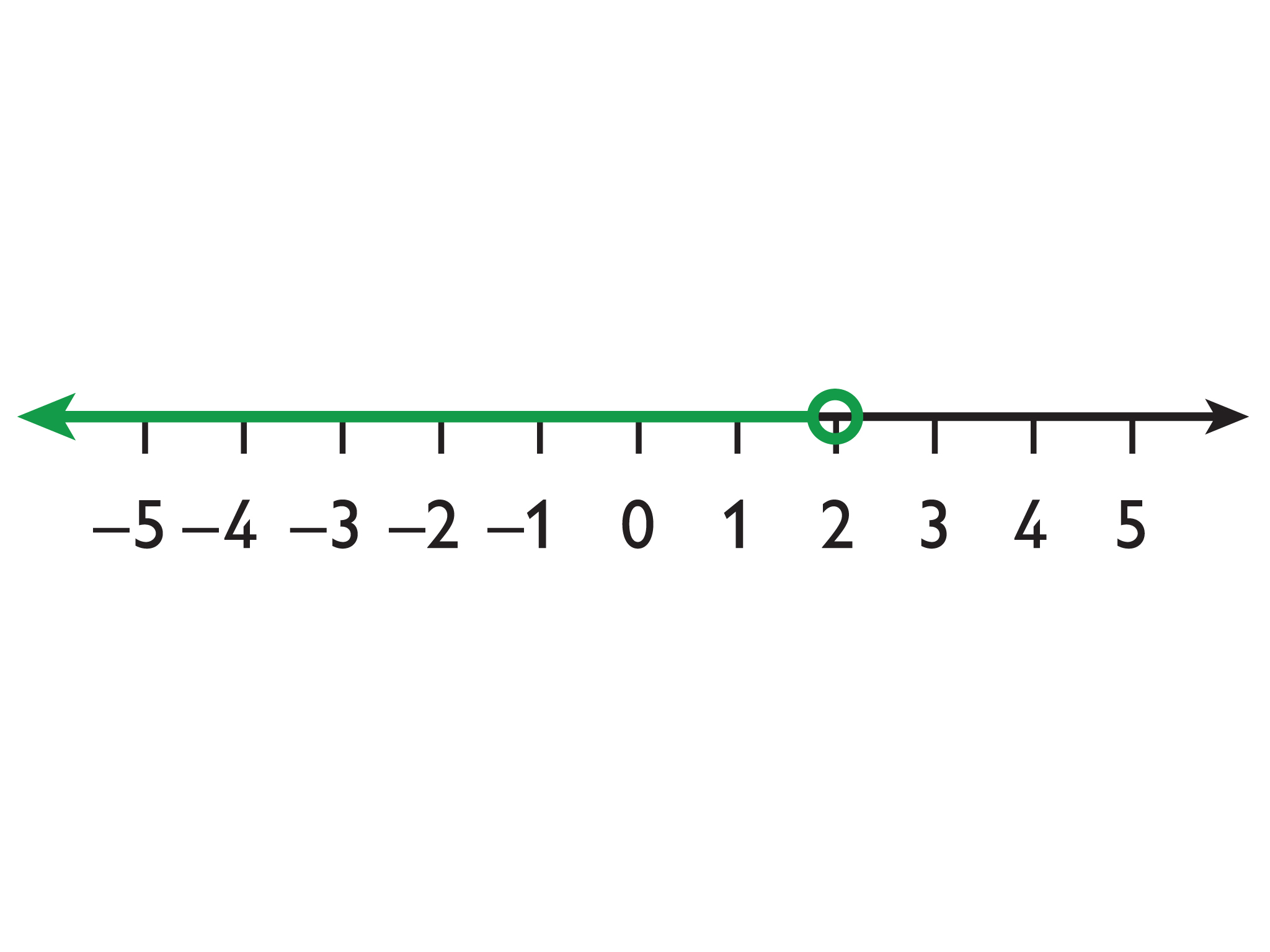The solutions to x > a are represented on the number line with an arrow pointing to the right from an open circle at a.

Example: x > 2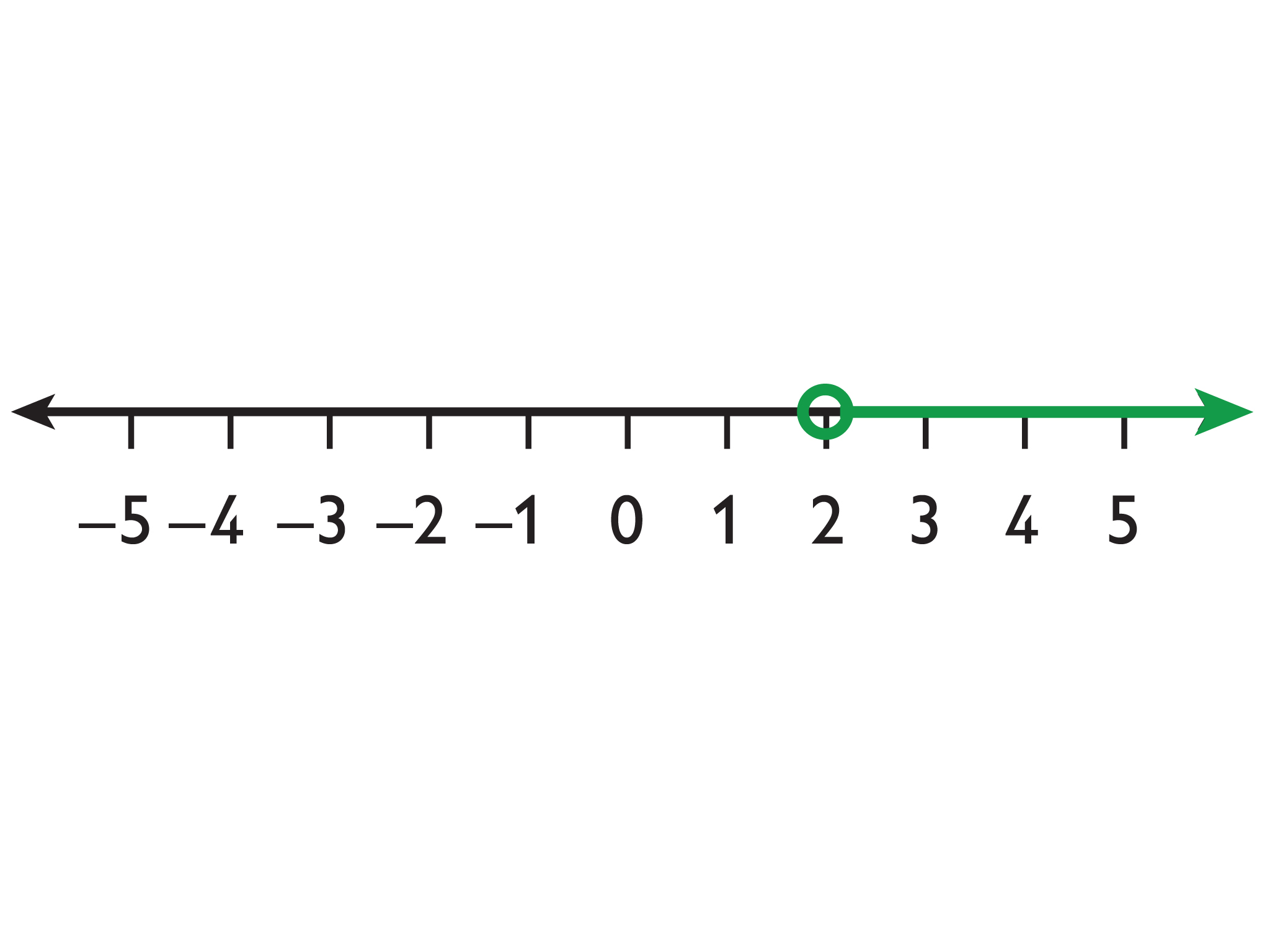The solutions to xa are represented on the number line with an arrow pointing to the left from a closed circle at a.

Example: x ≤ 2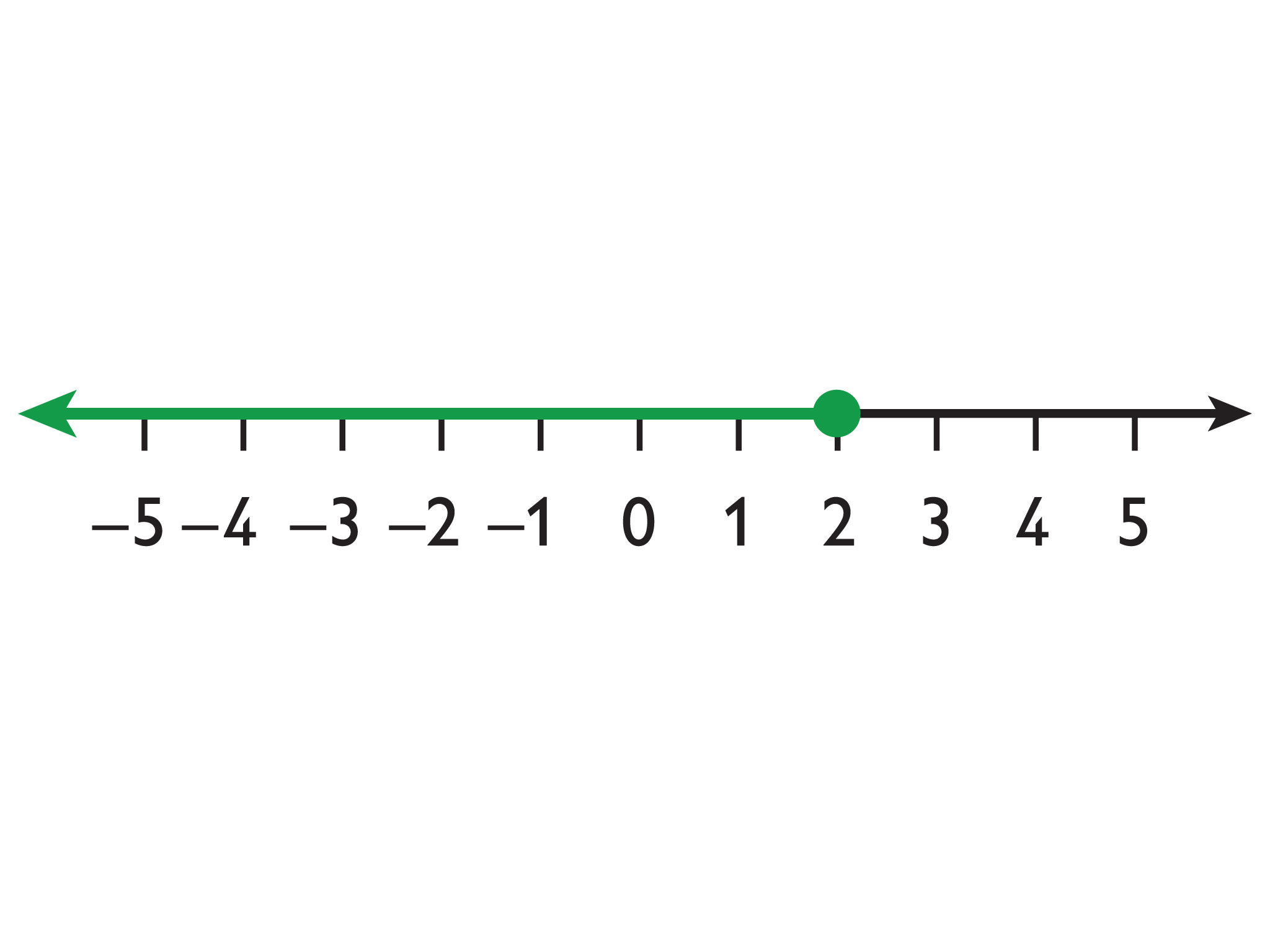The solutions to xa are represented on the number line with an arrow pointing to the right from a closed circle at a.

Example: x ≥ 2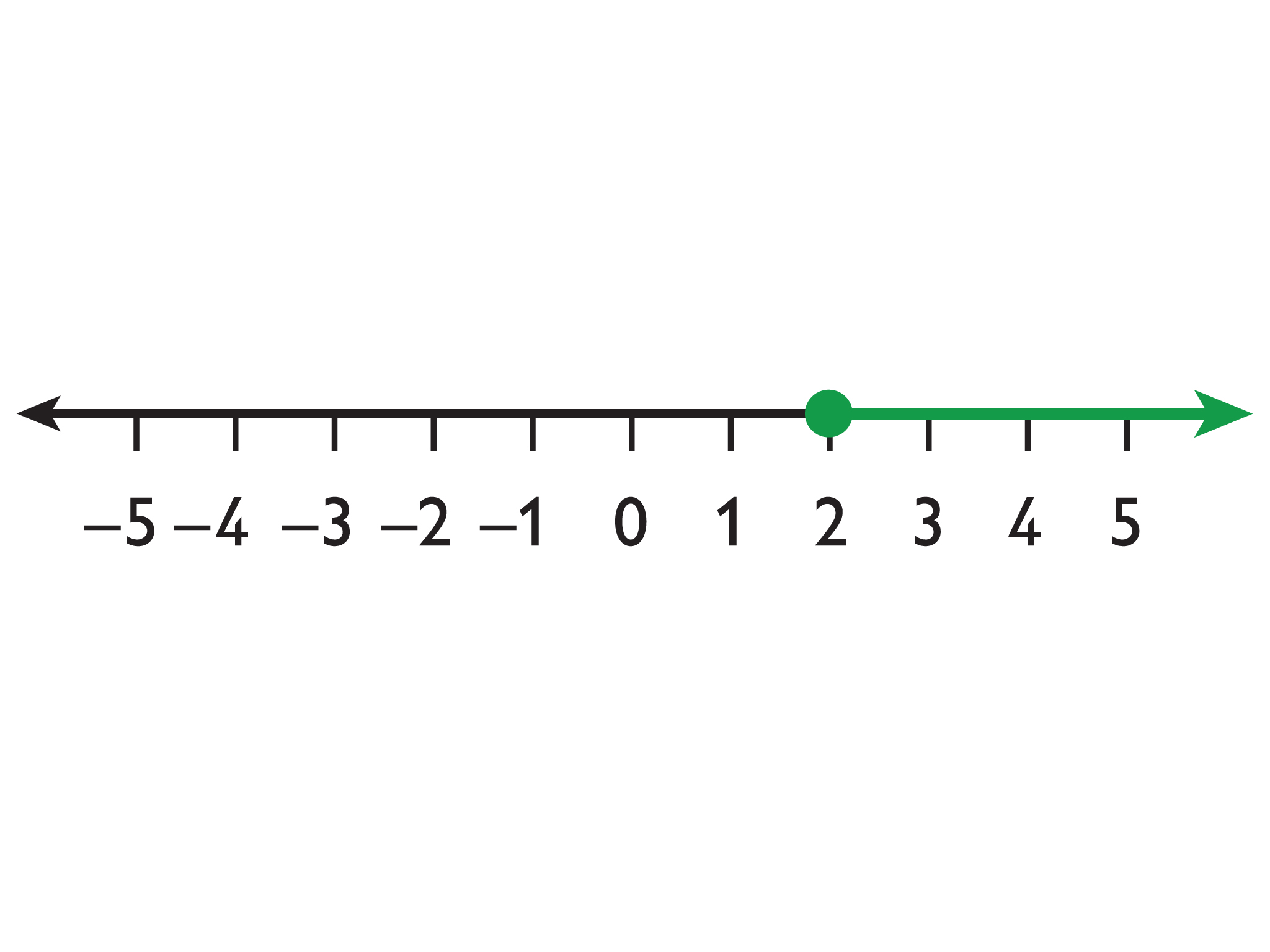# Goals and Learning Objectives

• Represent an inequality on a number line and using words.
• Understand that inequalities have infinitely many solutions.

# Lesson Guide

Have students work in pairs to match the inequalities with their representations on the number line and to write the inequality in words.

Share the inequalities from the Opening and the correct corresponding number line graphs with the students so they can easily reference them in the future.

ELL: Learning new ways of graphing inequalities can be a challenge for ELLs. Preview and/or pre-teach the new concepts in this lesson. Promote connections to students' background knowledge and to previous lessons in this unit.

# Mathematics

Students will use reasoning to match the number lines with the correct inequalities. They will need to reason that the open circle means that the number is not included in the inequality and the closed circle indicates that it is included. Do not provide this information for the students. If the class does not all agree on this convention initially, allow students to defend their positions until all see why this convention makes sense.

• x < 2: B. "x is less than 2."
• x > 2: C. "x is greater than 2."
• x ≥ 2: D. "x is greater than or equal to 2."
• x ≤  : A. "x is less than or equal to 2."

# Match Inequalities to Graphs

• Match each inequality to a number line.
• Write the inequality in words in the space provided; for example, "x is less than 5."

INTERACTIVE: Match Inequalities to Graphs

# Lesson Guide

Discuss the Math Mission. Students will represent inequalities on a number line and find at least one value that makes the inequality true.

## Opening

Represent inequalities on a number line and find at least one value that makes the inequality true.

# Lesson Guide

Have students work in pairs on the problems in Tasks 3 and 4. Identify any student misconceptions to address in Ways of Thinking.

SWD: Circulate through your classroom before your students share. Help students to identify ideas from their notes and thoughts that are appropriate to contribute during this portion of the Ways of Thinking discussion.

# Mathematical Practices

Mathematical Practice 3: Construct viable arguments and critique the reasoning of others.

Listen for student conversations about whether rules or conventions apply the same way to negative values as they do to positive values.

Mathematical Practice 6: Attend to precision.

Listen for student conversations about whether the circles should be open or closed.

Listen for student conversations about which direction the arrows should face.

# Interventions

Student does not understand how to use the interactive.

Student struggles with negative values and the direction of arrows.

• Is the number to the left greater than or less than the number to its right?

Student is confused about the conventions for using open and closed circles on the number line.

• Look back at the answers to the Opening.
• How are the math conventions connected to language: greater than or less than, and greater than or equal to or less than or equal to?

Student does not know how to find one value that makes the inequality true.

• Look at the open (or closed) circle. Does that number make the inequality true?
• Will the numbers that make the inequality true be to the left or right of the open (or closed) circle?

1.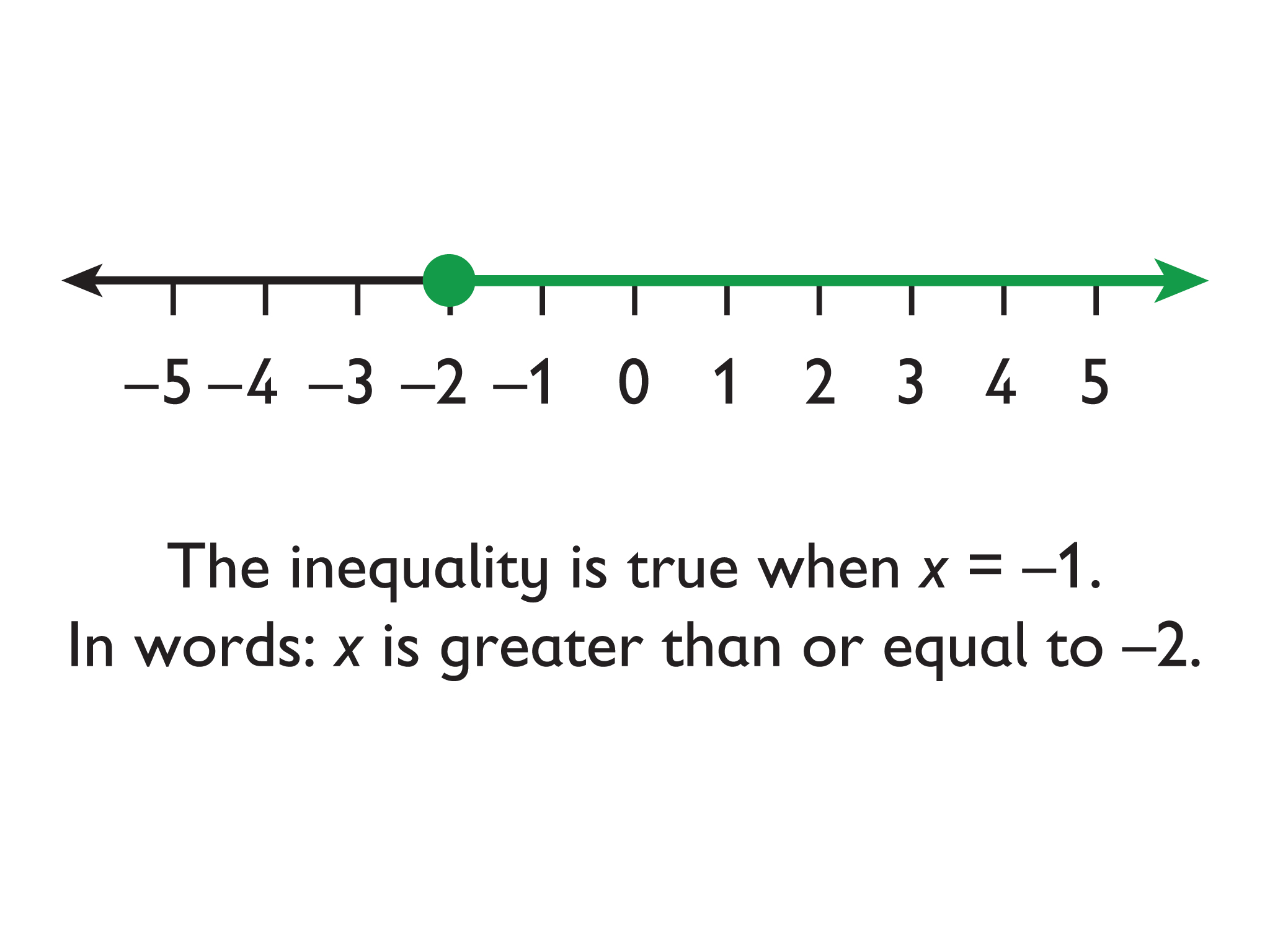2.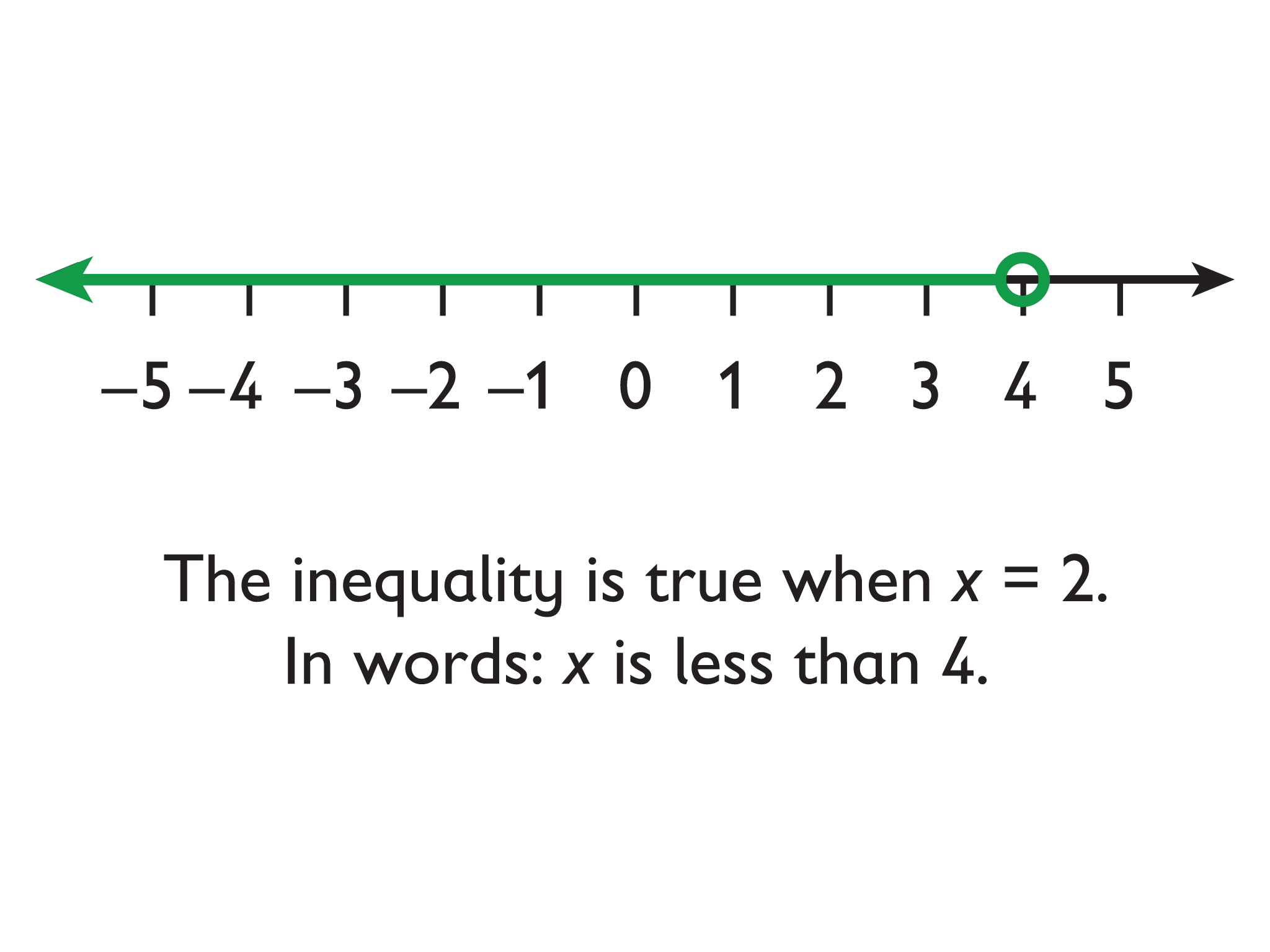3.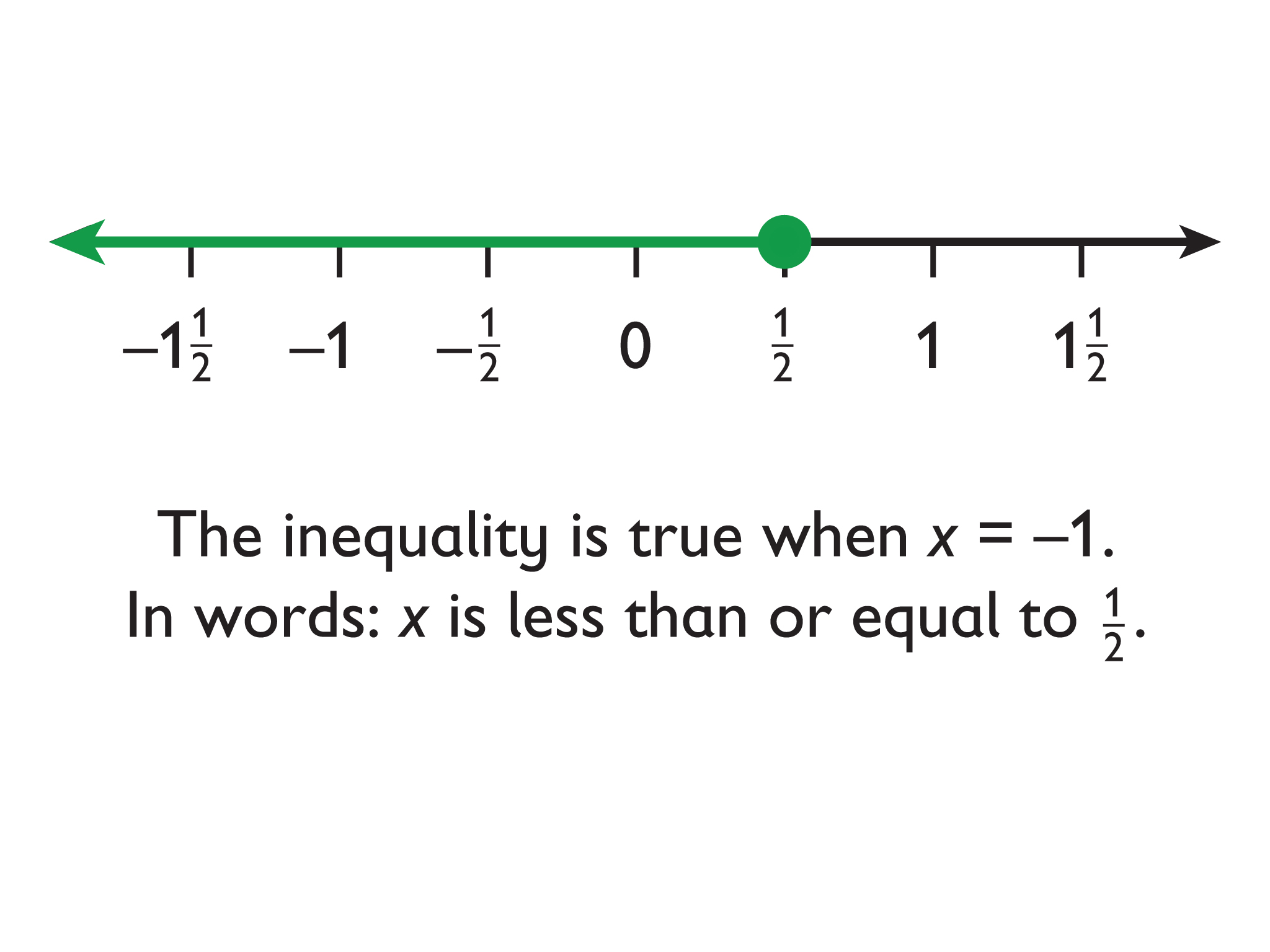4.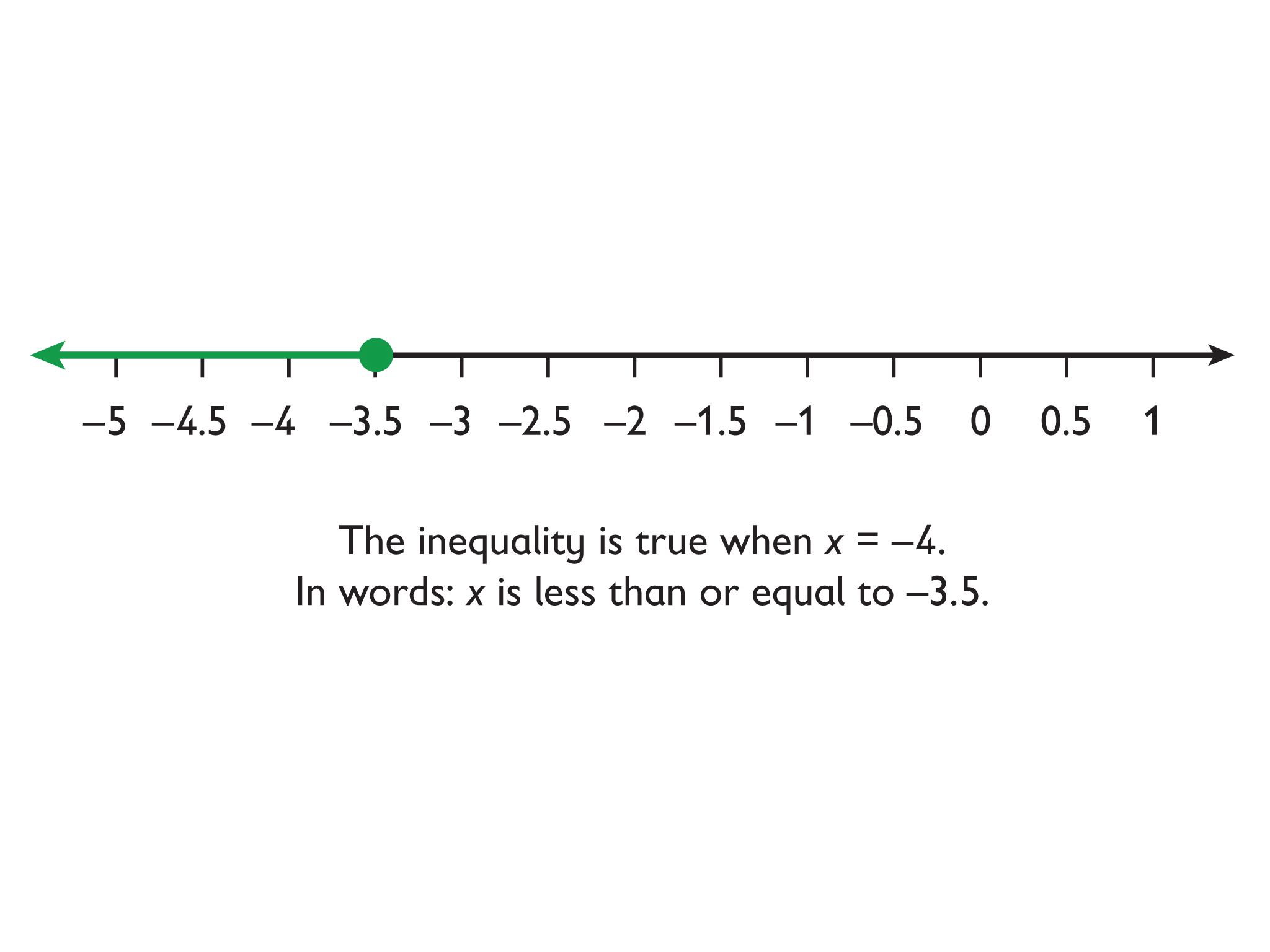5.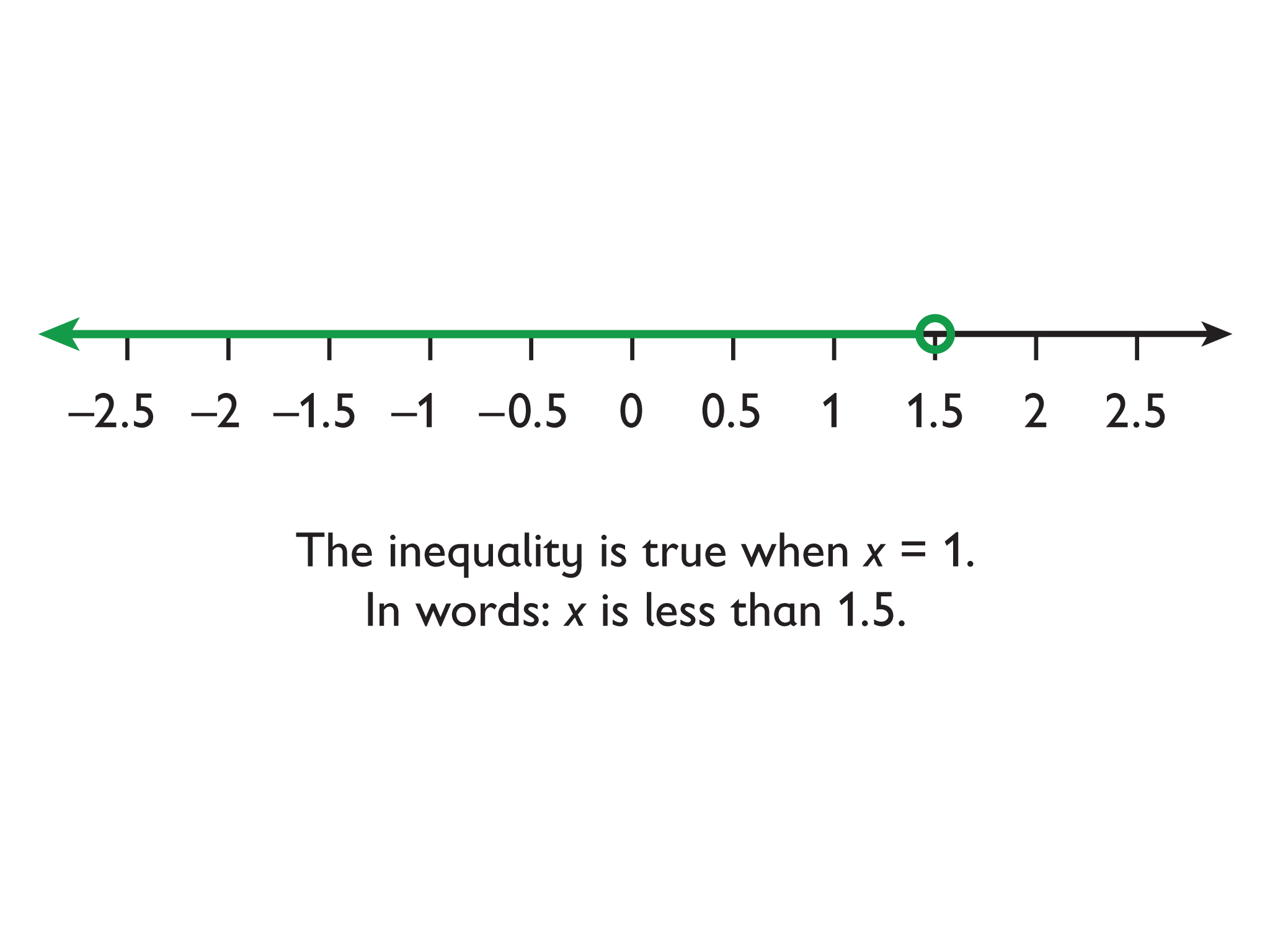# Inequalities and Number Lines

For each inequality do the following.

• Represent the inequality on a number line using the number line interactive.
• Write one value that makes the inequality true.
• Write the inequality using words.
1. x ≥ –2
2. x < 4
3. 12 ≥ x
4. x ≤ −3.5
5. x < 1.5

INTERACTIVE: Inequalities and Number Lines

## Hint:

• Where is the number located on the number line?
• Should the starting point be a closed circle or an open circle?
• What is one value that makes the inequality true? Is that value shaded on the number line?

# Lesson Guide

Watch for students who:

• Cannot write a real-world situation to represent the number line.

# Mathematical Practices

Mathematical Practice 1: Make sense of problems and persevere in solving them.

Look for a variety of real-world scenarios.

Mathematical Practice 3: Construct viable arguments and critique the reasoning of others.

Listen for student conversations about whether rules or conventions apply the same way to negative values as they do to positive values.

• Children must be at least 5 years old to attend the story hour.
• Four books cost $22 … • A purple paint is mixed in the ratio of … Student's word problem does not match the equation given. • Work backwards. Read the word problem you wrote. Write an equation that represents it. Does it match the equation you were given? # Answers 1. $\begin{array}{c}\\ x+5-5=17-5& \text{Addition property of equality}\\ x=12& \end{array}$ Word problems will vary. Possible answer: A shirt costs$5 more than a pair of shorts. The shirt costs $17. How much does the pair of shorts cost? Answer: The pair of shorts costs$12.

2. $\begin{array}{cc}\frac{1}{4}\cdot 4x=\frac{1}{4}\cdot 22& \text{Multiplication property of equality}\\ x=5\frac{1}{2}& \end{array}$

Word problems will vary. Possible answer: Four books cost $22. Each costs the same amount. How much does one book cost? Answer: One book costs$5.50.

3. $\begin{array}{c}\\ \frac{x}{6}\cdot 6=\frac{5}{8}\cdot 6& \text{Multiplication property of equality}\\ x=3\frac{3}{4}& \end{array}$

Word problems will vary. Possible answer: A purple paint is mixed in the ratio of 5 parts blue to 8 parts red. How much blue paint should be mixed with 6 cups of red paint to get the same shade of purple? Answer: You should mix 3$\frac{3}{4}$ cups of blue paint.

# Solve and Use Equations

Complete this Self Check by yourself.

For each equation do the following.

• Solve the equation. Name the property of equality you use and show each step.
• Write and solve a word problem that each equation could represent.
1. x + 5 = 17
2. 4x = 22
3. $\frac{x}{6}=\frac{5}{8}$